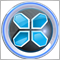# Simple code is not executing trades on crossing of exponential moving averages95

Hello all,  I have a very simple code that uses exponential moving averages at 2 lengths.  When the shorter length crosses above the other then a long position is taken.  Sometimes it works.  That is ,when there is an obvious crossing I can see on the chart a long position is taken but at other obvious crossings a long position is not taken.  Do you think this is related to the code or is it possibly a computer speed/processor issue?  Any hints would be appreciated.

```      double EMA11 = iMA_(Symbol(), Aggregation, EMAlength1, 0, MODE_EMA, PRICE_CLOSE, 1);
double EMA21 = iMA_(Symbol(), Aggregation, EMAlength2, 0, MODE_EMA, PRICE_CLOSE, 1);
double EMA12 = iMA_(Symbol(), Aggregation, EMAlength1, 0, MODE_EMA, PRICE_CLOSE, 2);
double EMA22 = iMA_(Symbol(), Aggregation, EMAlength2, 0, MODE_EMA, PRICE_CLOSE, 2);

//Long EMA approach
if (EMA11>EMA21)
if (EMA12<EMA22)
golongnew = true;
```28618

dclark24:

Hello all,  I have a very simple code that uses exponential moving averages at 2 lengths.  When the shorter length crosses above the other then a long position is taken.  Sometimes it works.  That is ,when there is an obvious crossing I can see on the chart a long position is taken but at other obvious crossings a long position is not taken.  Do you think this is related to the code or is it possibly a computer speed/processor issue?  Any hints would be appreciated.

Hi,

So sometime below condition may happen:

```if (EMA11==EMA21)
if (EMA12<EMA22)

or

if (EMA11>EMA21)
if (EMA12==EMA22)
```

So it could be :

```if (EMA11>=EMA21)
if (EMA12<EMA22)

or

if (EMA11>EMA21)
if (EMA12<=EMA22)```

You can print the values which you see the crossing is happened,and see the exact condition.19441

```double aPrev = …, aCurr = …,
bPrev = …, bCurr = …;
bool   wasUp = aPrev > bPrev,
isUp = aCurr > bCurr,
isCross = isUp != wasUp;```
To add comments, please log in or register